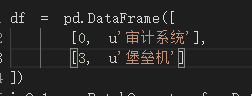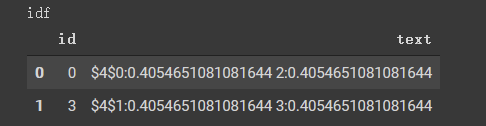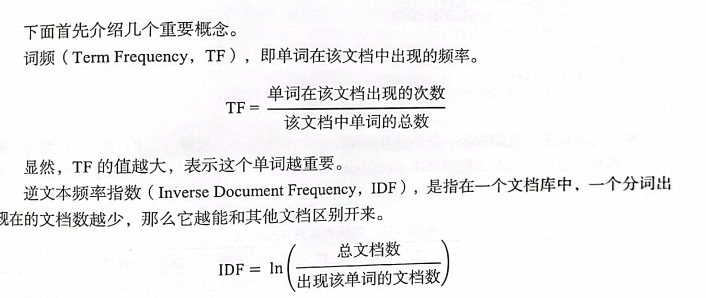# 第21章 文本分析

## 答疑

### 1、IDF的计算问题

【问题】

 idf计算是不是错了？idf计算结果用底数为e或2都不是这个结果【答复】

double idf = Math.log((1.0 + docCnt) / (1.0 + df));

System.out.println(Math.log((1.0+2)/(1.0+1)));

【问题】

【答复】

### 3、英文的切分

【问题】

【答复】

```new MemSourceBatchOp(new Object[] {"example"}, "txt")
new RegexTokenizerBatchOp()
.setGaps(false)
.setPattern(".")
.setSelectedCol("txt")
)
new NGramBatchOp()
.setSelectedCol("txt")
.setOutputCol("txt_2")
.setN(2)
)
new NGramBatchOp()
.setSelectedCol("txt")
.setOutputCol("txt_3")
.setN(3)
)
.print();```

```txt|txt_2|txt_3
---|-----|-----
e x a m p l e|e_x x_a a_m m_p p_l l_e|e_x_a x_a_m a_m_p m_p_l p_l_e```

【问题】

【答复】